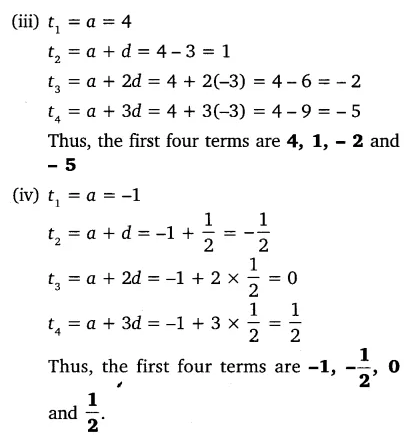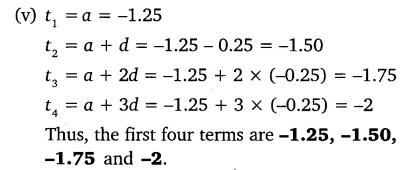# Write first four terms of the AP, when the first term a and the common difference

Write first four terms of the AP, when the first term a and the common difference d are given as follows:
(i) a = 10, d = 10
(ii) a = -2, d = 0
(iii) a = 4, d = -3
(iv) a = -1, d = 1/2
(v) a = -1.25, d = -0.25